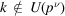International
Tables for
Crystallography
Volume B
Reciprocal space
Edited by U. Shmueli

International Tables for Crystallography (2010). Vol. B, ch. 1.3, p. 56   | 1 | 2 |

## Section 1.3.3.2.3.2. N a power of an odd prime

G. Bricognea

aGlobal Phasing Ltd, Sheraton House, Suites 14–16, Castle Park, Cambridge CB3 0AX, England, and LURE, Bâtiment 209D, Université Paris-Sud, 91405 Orsay, France

#### 1.3.3.2.3.2. N a power of an odd prime

| top | pdf |

This idea was extended by Winograd (1976, 1978) to the treatment of prime powers, using the cyclic structure of the multiplicative group of units. The latter consists of all those elements ofwhich are not divisible by p, and thus haselements. It is cyclic, and there exist primitive roots g modulosuch thatTheelements divisible by p, which are divisors of zero, have to be treated separately just as 0 had to be treated separately for.

When, thenwith. The resultsare p-decimated, hence can be obtained via the-point DFT of the-periodized data Y:withWhen, then we may writewherecontains the contributions fromandthose from. By a converse of the previous calculation,arises from p-decimated data Z, hence is the-periodization of the-point DFT of these data:with(the-periodicity follows implicity from the fact that the transform on the right-hand side is independent of).

Finally, the contributionfrom allmay be calculated by reindexing by the powers of a primitive root g modulo, i.e. by writingthen carrying out the multiplication by the skew-circulant matrix core as a convolution.

Thus the DFT of sizemay be reduced to two DFTs of size(dealing, respectively, with p-decimated results and p-decimated data) and a convolution of size. The latter may be `diagonalized' into a multiplication by purely real or purely imaginary numbers (because) by two DFTs, whose factoring in turn leads to DFTs of sizeand. This method, applied recursively, allows the complete decomposition of the DFT onpoints into arbitrarily small DFTs.

### References

Winograd, S. (1976). On computing the discrete Fourier transform. Proc. Natl Acad. Sci. USA, 73, 1005–1006.
Winograd, S. (1978). On computing the discrete Fourier transform. Math. Comput. 32, 175–199.